# 9. PTM2, C2 – Linear Regression

## 9.1 – Introduction to Linear Regression

The previous chapter laid down a basic understanding of a straight line equation. To keep things simple, we took a very basic example to explain how two variables can be related to each other. Needless to say, the examples were selected in a way that casual eyeballing could reveal the relationship. Towards the end of the chapter we posted a table containing two arrays of numbers – the task was to figure out if there was a relationship between the two sets of numbers, if yes, what how could one express the relationship in the form of a straight line equation. More precisely, what was the intercept and constant?

We will figure how to establish a relationship in this chapter and move closer towards the relative value trading technique. For convenience, let me post the table with the two number arrays once again –

X Y
10 3
12 6
8 4
9 17
20 36
18 22

Clearly, casual eyeballing does not reveal any information about the relationship between the two sets of numbers. Maybe it does, if you are a mutant, but for a mere mortal like me, it does not work.Under such circumstances, we rely upon a technique called the ‘Linear Regression’. Linear regression is a statistical operation wherein the input is an array of two sets of numbers and the output contains many different parameters, including the intercept and constant needed for constructing the straight line equation.

To perform the linear regression operation, we will depend on the good old Excel.  Here is the step by step guide to perform a simple linear regression on two arrays of numbers. Be prepared to see a lot of screenshots and instructions ☺

Step 1Install the Plugin

Open a fresh excel sheet and insert the values of X & Y as seen in the above table. I’ve done the same as shown below –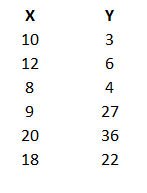This is our data set. Do remember, Y is the ‘Dependent’ variable whose value depends on the independent variable X. Both X and Y will be the input variables for the linear regression operation.

On the excel sheet, click on the Data ribbon as highlighted in red, shown below –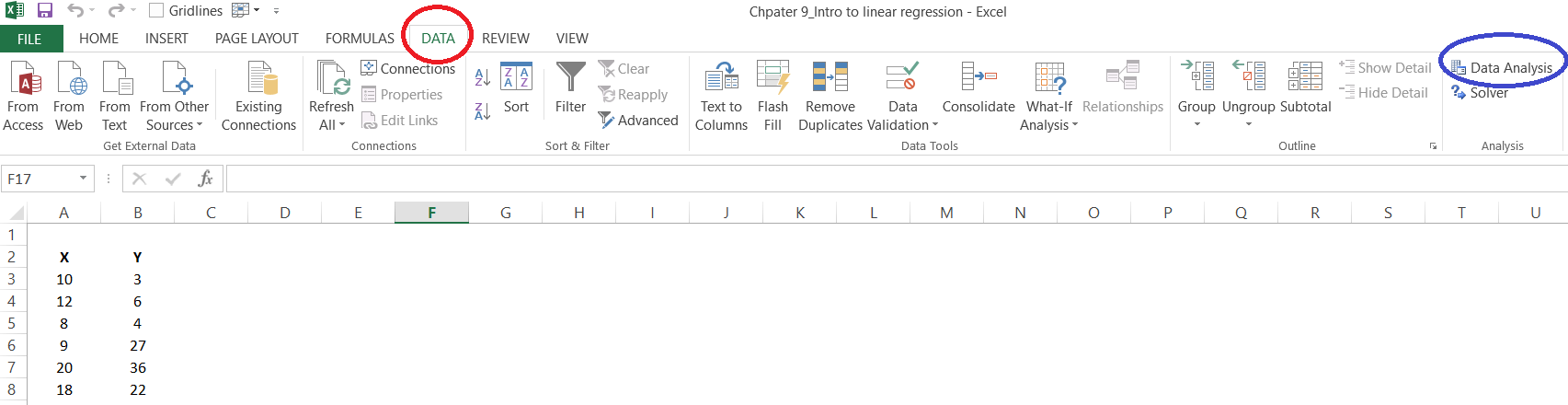The data ribbon will now show you the ‘Data Analysis’, option. This is highlighted in blue. Now, some of you may not see this option, if yes, don’t panic. I’ll tell you what needs to be done.

Click on ‘File’ –This will open up a new window, and on your left-hand side panel, you will see an option to select ‘option’ –Click on the Options, and you will see a bunch of general options to work with. On the left-hand panel, select ‘Add-Ins’, click on it and then click on the ‘Analysis Tool pack’. Then click on ‘Go’, and finally on ‘Ok’. With this, you’d essentially added the ‘Data Analysis’ option to the data ribbon.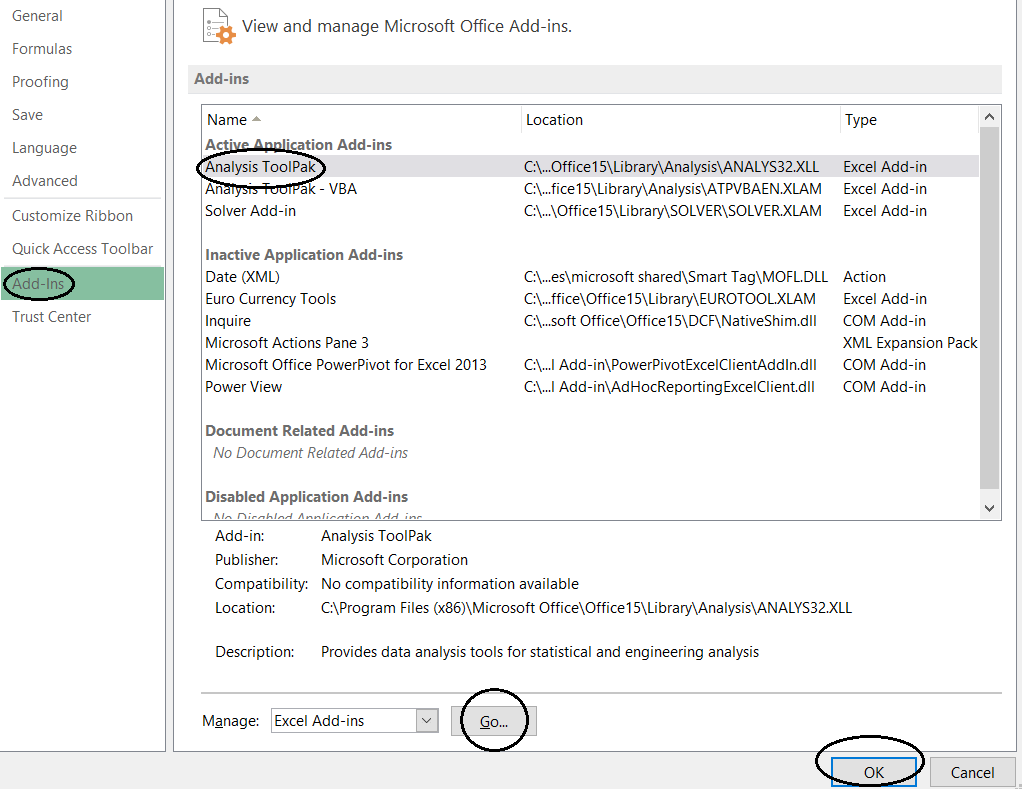Close the excel sheet and restart your system and you are good to roll.

Step 2 – Enter the values

So we proceed further based on the assumption that your excel sheet has the data analysis pack. The next step is to invoke the linear regression function within the data analysis pack. To do this, click on the ‘Data’ ribbon, and select the Data Analysis. This will open up a pop-up, which will have a list of statistical operations which you can perform on data sets. Select the one which says ‘Regression’.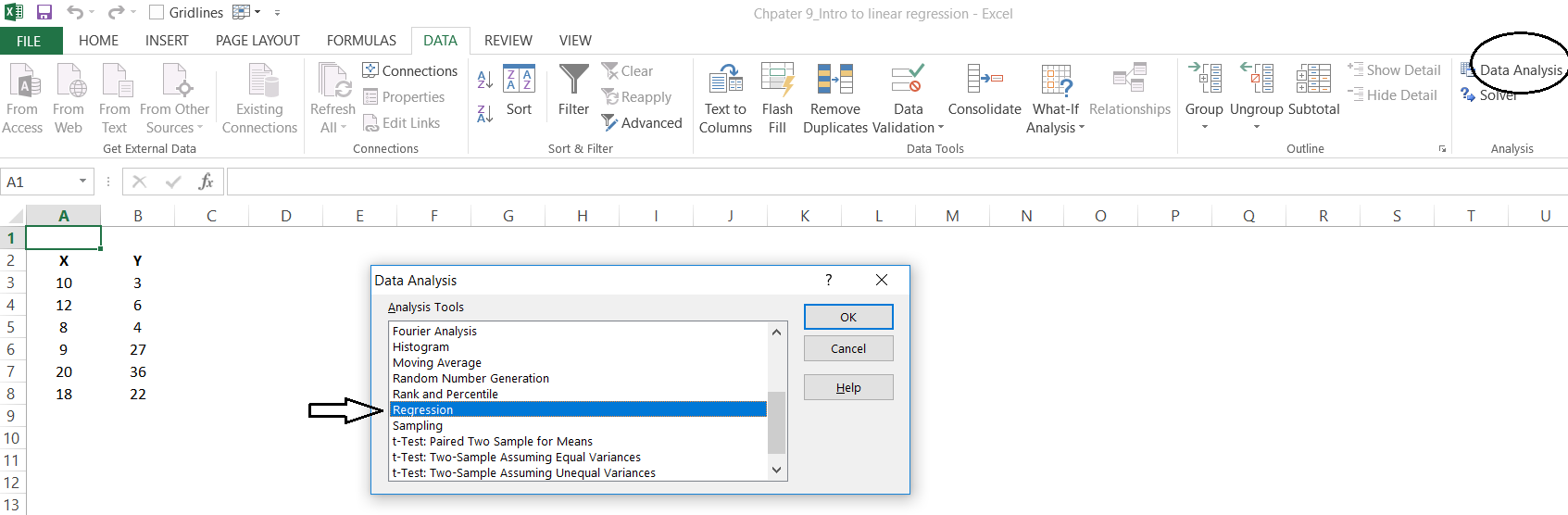Select regression and click ok, you will see the following pop up –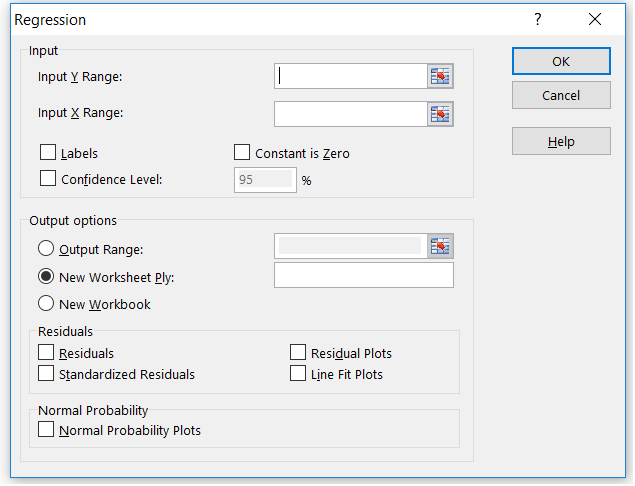As you can see, there are a bunch of fields here. I’d suggest you pay attention to the first section, which is the input section. There are two fields here – ‘Input Y Range’ and ‘Input X Range’. As you may have imagined, Y is for the dependent variable and X is for the dependent variable.

This is where we feed in the X and Y series data. To do that, click on the input channel and select Y and X range –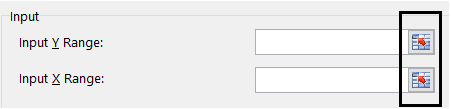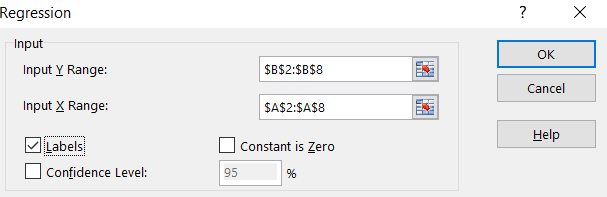Also, please notice that I’ve checked the label box, this indicates that the first cell value i.e A2 and B2 contain the series label i.e X & Y respectively.

I’d suggest you ignore the other input values for now.

On the output side, ensure you’ve clicked the following –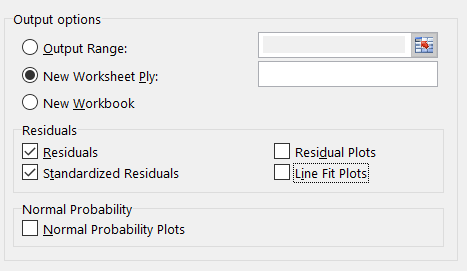Selecting ‘New worksheet’, ensures that the output data is printed on a new worksheet. I’ve also clicked on two other variables called – Residuals and Standardized Residuals. I will talk about these two variables at a later point. For now, just ensure they are selected.

With this, you are good to perform the linear regression operation. Click on the ‘Ok’ button which is available in the right-hand top corner.

Excel will now take these inputs and perform the linear regression operation, the results will be posted in a new sheet within the same workbook.

## 9.2 – Linear Regression Output

So here is how the linear regression output looks and as expected, the summary of the output is presented in a new sheet.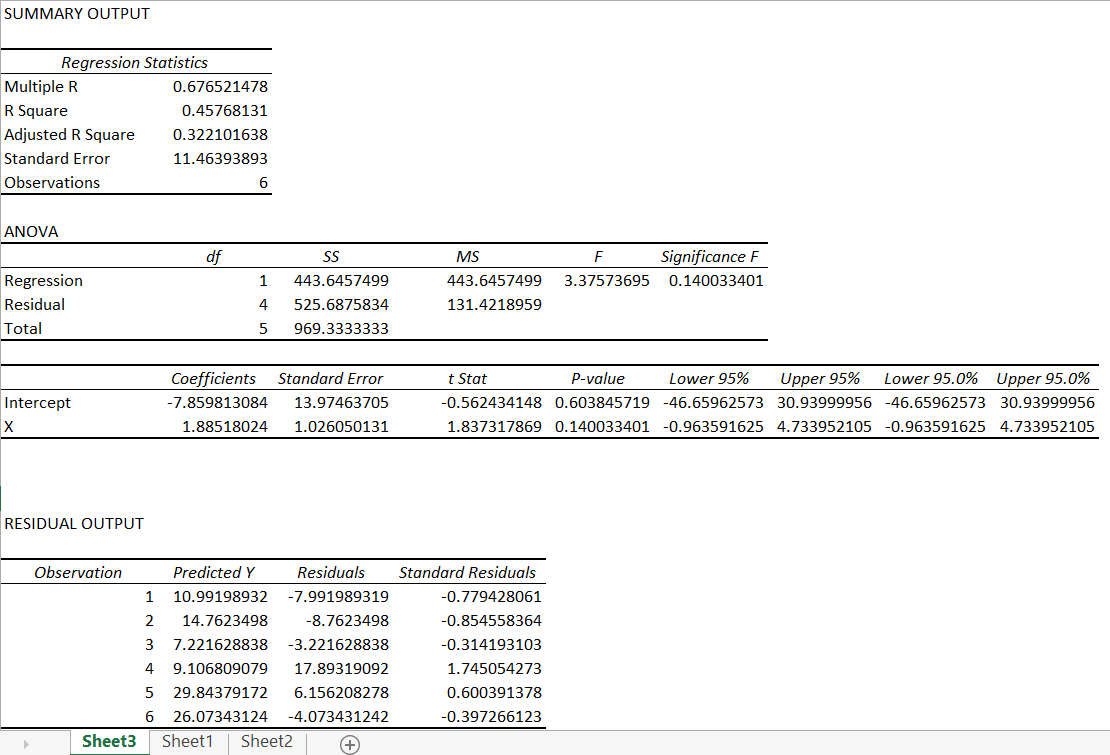Agreed, the summary output is quite scary at the first glance. It has lots and lots of information. We will unravel this output in bits and pieces as we proceed.

For now, let’s concentrate on finding our slope and intercept. I’ve highlighted this for you in the below snapshot –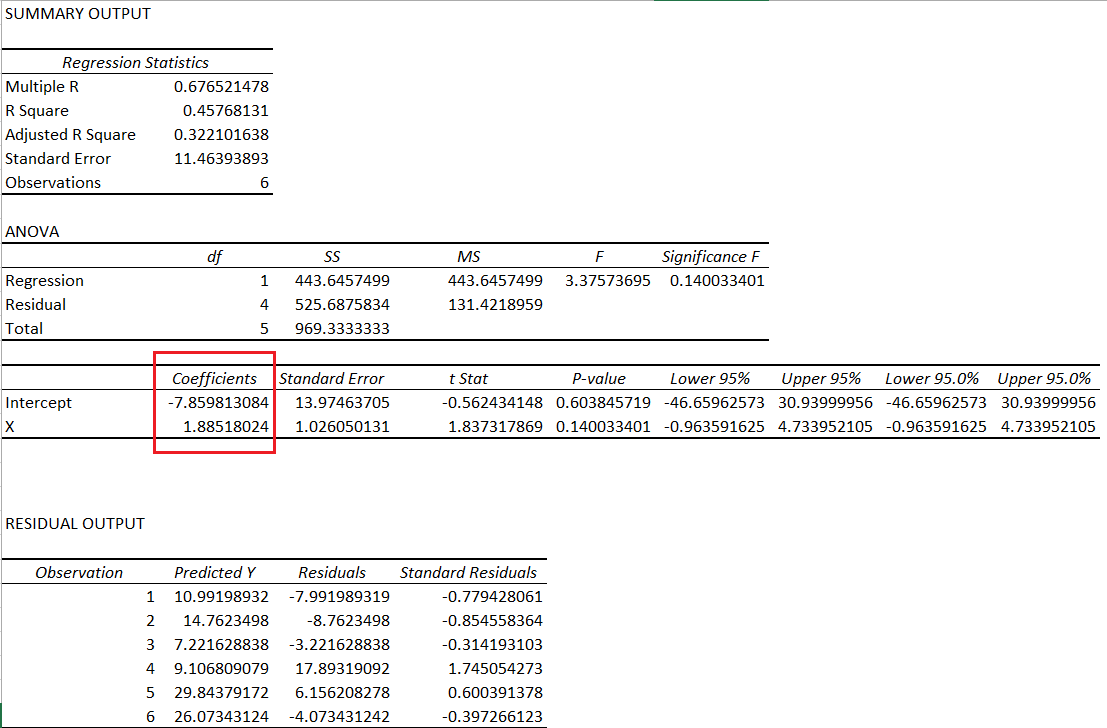The data points highlighted in red contains the coefficients we are looking for i.e the intercept (or constant) and the slope (denoted by x).

Some of you may be confused with the slope being represented by x, I understand its misleading, it would have been best if it was M instead of x as it would match the straight-line equation, but then I guess we will have to live with x for slope.

So,

• Slope of the equation = 1.885
• Intercept (or constant) = -7.859813.

Given this, the straight-line equation for the arbitrary set of data is –

y = 1.885*x + (-7.859813) or

y = 1.885*x – 7.859813

So what does this really mean?

Well, if you recollect from the previous chapter, this equation essentially helps us predict the value of y or the dependent variable for a certain x. Let me repost the table here for the sake of convenience –

 X Y 10 3 12 6 8 4 9 17 20 36 18 22 15 ??

I’ve added a new data point for x here i.e 15, now using the slope and intercept, we can predict the value of y. Let’s do that –

y = 1.885 * 15 – 7.859813

= 28.275 – 7.859813

= 20.415

So, if x is 15, then most likely, the predicted value of y is 20.415.

How accurate is this prediction, you may ask?

Well, it’s not accurate. It is only an estimation. For example, consider the value of x is 18 (refer to the last but one data point), then according to the straight line equation, the value of y should be –

y = 1.885*18 – 7.859813

= 33.93 – 7.859813

= 26.07019

However, the actual value of y is 22.

This leads us two values of y –

1. Predicted value of y via the straight line equation
2. Actual value of y

The difference between the two values of y is called the residuals. For example, the residual for y (difference between actual and predicted y), when x = 18 is

26.07019 – 22

= 4.070187

The summary output when you perform linear regression also contains the residuals, I’ve highlighted the same in the snapshot below –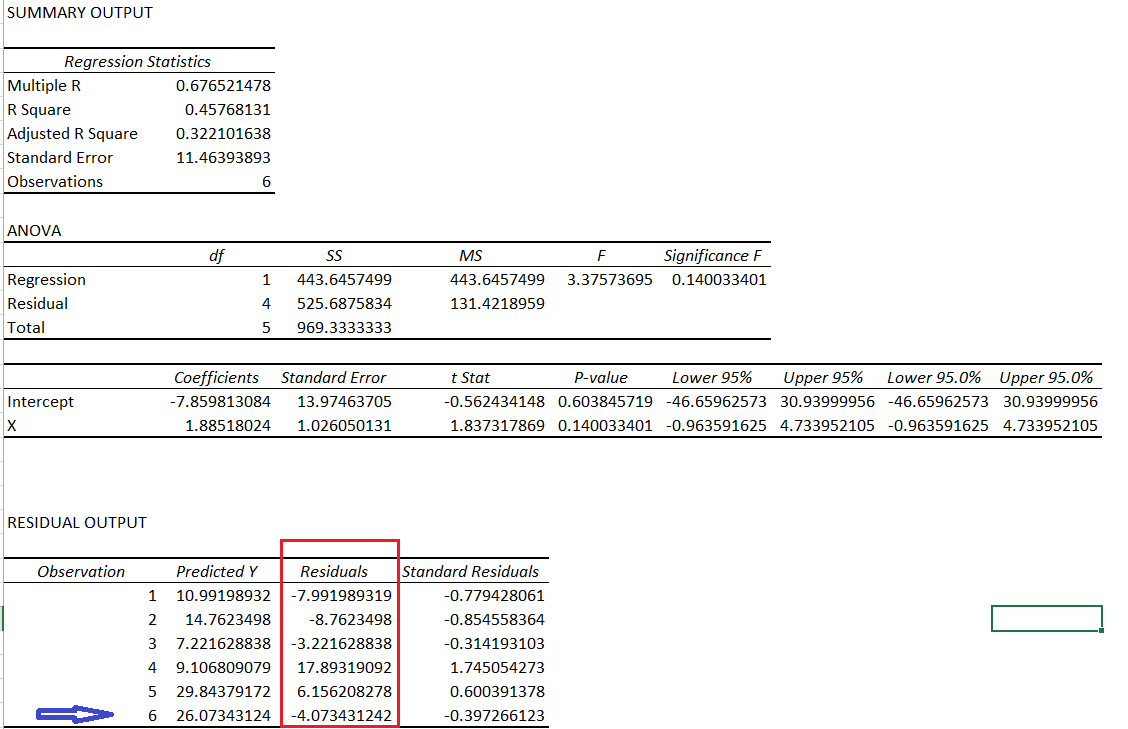I’ve also highlighted the residual when x = 18, which is what we calculated above.

To give you a heads up – the bulk of the focus for carrying out the relative value trade depends on the residuals. Stay tuned!

### Key takeaways from this chapter

1. Linear regression is a statistical operation which helps you construct a straight line equation
2. Linear regression can be performed on excel. One needs to install the excel plugin to perform linear regression
3. Amongst many other output variables, linear regression gives out the values of the slope and intercept
4. With the help of the slope and intercept, one can predict the value of y
5. The difference between actual y and predicted y is called the residual
6. The residual is also a part of the output summary

1.kmayank says:

I have been waiting for it. Thanks:)

•Karthik Rangappa says:

Happy learning 🙂

•Jay says:

Can you develop charting tool to compare two stocks like techpaisa.com. Is there any other website

•Karthik Rangappa says:

You can compare two stocks on Kite3, have you tried that yet?

•rohit jain says:

comparing two stocks in kite? can you share a link how to do this? Just joined zerodha…Will be interested in knowing about this.

•Faisal Mohammed says:

Comparing 2 scrips on Kite is explained in this TradingQ&A post

•rohit jain says:

Thank you. i will search

•Karthik Rangappa says:

Good luck!

2.Deepu says:

Fantastic Karthik!!!

I eagerly wait for your new chapters like a school kid and check the varsity everyday. Hope to have the next chapter soon:)

Thanks for sharing the knowledge in such a lucid manner.

Thanks.

•Karthik Rangappa says:

Happy to note that, Deepu 🙂 Motivates me to write more and faster 🙂

3.sharath says:

•Karthik Rangappa says:

Will be up once the module is completed.

4.Rajaram says:

Dear Karthik,

The sense that your articles are providing to understand the mindless market is quite great, I have never been fan of boring statistics 🙂 but the applications of the statistical methods here, has made me think differently. I guess this can be attributed to the real world application of statistics instead of the theoretical education that I was used to.

A stupendous work, I hope you continue to be a beacon for all of us.

Regards
Rajaram

P.S: I think there is a typo “As you may have imagined, Y is for the dependent variable and X is for the dependent variable”
Shouldn’t X be the independent variable.

•Karthik Rangappa says:

Thanks for the kind words, Rajaram. I’m really happy to note that you’ve liked the content here 🙂

Yes, that’s a typo, will fix that, thanks for pointing out 🙂

•Priya says:

Karthik – typo not fixed yet 🙂

5.Rohit says:

You have not mentioned about the use of linear regression.

•Karthik Rangappa says:

Next chapter 🙂